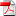# Fast multivariate empirical cumulative distribution function with connection to kernel density estimation - Nicolas Langrené & Xavier Warin

1
Juil

This paper revisits the problem of computing empirical cumulative distribution functions (ECDF) efficiently on large, multivariate datasets. Computing an ECDF at one evaluation point requires O(N) operations on a dataset composed of N data points. Therefore, a direct evaluation of ECDFs at N evaluation points requires a quadratic O(N^2) operations, which is prohibitive for large-scale problems. Two fast and exact methods are proposed and compared. The first one is based on fast summation in lexicographical order, with a O(NlogN) complexity and requires the evaluation points to lie on a regular grid. The second one is based on the divide-and conquer principle, with a O(N log(N)^((d−1)v1)) complexity and requires the evaluation points to coincide with the input points. The two fast algorithms are described and detailed in the general d-dimensional case, and numerical experiments validate their speed and accuracy. Secondly, the paper establishes a direct connection between cumulative distribution functions and kernel density estimation (KDE) for a large class of kernels. This connection paves the way for fast exact algorithms for multivariate kernel density estimation and kernel regression. Numerical tests with the Laplacian kernel validate the speed and accuracy of the proposed algorithms. A broad range of large-scale multivariate density estimation, cumulative distribution estimation, survival function estimation and regression problems can benefit from the proposed numerical methods.

•Laplacian_kernel